# Project 1: Electron Plasma Waves

Imported and adapted from the UCLA Plasma Simulation Group's JupyterPIC Notebooks

Before anything else, we need to change to the stored notebook's directory, which contains input files for the PIC simulator.

cd /notebooks/electron-plasma-wave-dispersion

The display of a prerendered graphic via Jupyter cell was replaced with a direct upload of the image file.

In this project, we are going to look at the dispersion relation for electron plasma waves.

The dispersion relation, $\omega(k)$, tells us the natural frequencies of oscillations for these waves, and the information contained in this function about the relationship between $\omega$ and $k$ can be used to determine the phase and group velocities of these waves. [There will be a subsequent notebook on wave velocities]

Take the following simple case:

• $\nabla \times \vec{E} = 0$ -- Longitudinal (electrostatic) waves

• $T_i = T_e = 0$ -- Cold plasma

• $\vec{B}_0 = 0$ -- Unmagnetized

The time derivative of Ampere's law in the unmagnetized case gives:

Using Euler's equation, $\partial \vec{v} / \partial t = (q/m)(\partial \vec{E} / \partial t)$,

and by using the plasma frequency definitions,

we have

This is our familiar harmonic oscillator equation.

In a hydrogen plasma with $m_i = 1836 m_e$, the frequency is dominated by the electron plasma frequency: $\omega_p^2 + \Omega_p^2 = \omega_p^2 [ 1 + m_e/m_i ] \approx \omega_p^2$, and in this case,

To Fourier Analyze the equation (and make it an algebraic equation to solve rather than a partial differential equation), we substitute in $\vec{E} = \vec{\bar{E}} e^{i\omega t}$, giving

This equation leads quite simply to the dispersion relation:

#
# Plotting w(k) = w_p
# Rather straightforward, since there is no dependence on k
#
import numpy as np
import matplotlib.pyplot as plt
%matplotlib inline
x = np.linspace(-25,25,100)
y = np.ones(100)
plt.plot(x,y)
plt.xlabel('k [$c/\omega_p$]'); plt.ylabel('$\omega$ (in units of $\omega_p$)'); plt.xlim(-25,25); plt.ylim(0,3)
plt.show()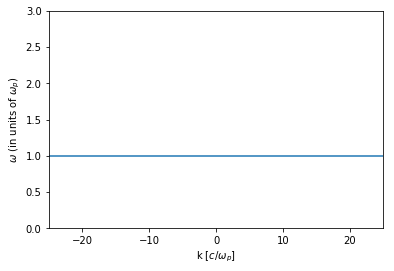The frequency of oscillation for waves under these plasma conditions is $\omega = \pm \omega_p$, and we see that there is no dependence on $k$.

There can be a spatial variation to the waves, because $\vec{\bar{E}}$ can be an arbitrary function of position, and we can have $\vec{E} = \vec{\bar{E}}(\vec{x})e^{i\omega_p t}$ as long as we satisfy our initial assumption that $\nabla \times \vec{E} = 0$.

Since there is no dependence on $k$, at each location the fields oscillate at $\omega_p$ independently of the other positions.

It is possible to phase these oscillations into a wave $\vec{E} = \bar{E}e^{i(kx-\omega_p t)}\hat{x}$ where $k$ is arbitrary.

$v_{\phi} = \omega/k = \omega_p/k$ and can take on arbitrary values depending on $k$.

$v_g = \partial\omega/\partial k = 0$ and the waves cannot transport any information.

This case is interesting but rather basic. Once we introduce a temperature to the plasma, there is a frequency dependence on the wavenumber.

## 1. Electron plasma waves in a warm plasma

We'll still assume:

• $\nabla \times \vec{E} = 0$ -- Longitudinal (electrostatic) waves

• $\vec{B}_0 = 0$ -- Unmagnetized

but now

• $T_e \neq 0$ -- Warm fluid

And from the start we'll assume that the ions do not move.

Here the time derivative of Ampere's law in the unmagnetized case gives:

And using Euler's equation in a warm plasma now gives:

To get the wave equation for $\vec{E}$, we must find $n_1$ in terms of $E$. To do this we use Gauss' Law:

so

where we used the fact that $\nabla(\nabla \cdot \vec{E}) = \nabla^2 \vec{E}$ when $\nabla \times \vec{E} = 0$.

Now we can write the equation purely in terms of $\vec{E}$:

For high frequency waves there is no time for any heat loss and we use $\gamma = 3$, i.e., the adiabatic equation of state for these 1D longitudinal oscillations. $\gamma = 3$ also follows from a more rigorous kinetic treatment. We can also substitute in $\omega_p$ and $v_{the}$.

Fourier Analyzing with $\vec{E} = \vec{\bar{E}}e^{i(kx-\omega t)}$,

giving the dispersion relation:

#
# Plotting w(k) = sqrt[w_p^2 + 3 * v_the^2 * k^2]
# We assume here that w_p = 1.0 and v_the/c = 0.05
#
import numpy as np
import matplotlib.pyplot as plt
%matplotlib inline
x = np.linspace(-25,25,100)
y = np.sqrt(1 + 3 * 0.05**2 * x**2)
plt.plot(x,y)
plt.xlabel('k [$c/\omega_p$]'); plt.ylabel('$\omega$ (in units of $\omega_p$)'); plt.xlim(-25,25); plt.ylim(0,3)
plt.show()## 2. Simulations with a Particle-in-Cell Code

In this project, you will be simulating plasmas in which each plasma electron is initialized with positions (only in x, or what we call $x_1$) such that the density is uniform. The ions are initialized at the same positions but they have an infinite mass. Each electron is also initialized with velocities ($v_1$, $v_2$, $v_3$) or momentum ($mv_1$, $mv_2$, $mv_3$) from a Maxwellian in each direction. The particles then begin to move in the self-consistent fields that their current and charge density produce.

• The length of the plasmas is 50 $c/\omega_p$
• The simulation will run for a time 400 $1/\omega_p$.
• The simulation uses 50,000 particles.

You will be looking at plots of the electric field in the $x_1$ direction, $E_1$.

### 2.1. The following lines must always be executed before running anything else.

Reminder: Hit Shift+Enter to run a cell, or select the cell and click on the "Run" button in the top menu bar

import osiris
%matplotlib inline

## 3. Run a case in which $vth_1=vth_2=vth_3=0.02 c$.

# vth/c = 0.02
dirname = 'v02'
osiris.runosiris(rundir=dirname,inputfile='v02.txt')

After the simulation is finished, plot $E_1(x_1)$ at $t \approx 100$ (run the next cell).

• Do you see any evidence of a plasma wave or oscillation?
• Does the plot make sense?
dirname = 'v02'
osiris.field(rundir=dirname,time=100)Next, plot $E_1(t)$ at $x_1=5 c/\omega_p$ (i.e., at cell=100).

• Do you see any evidence of a plasma wave or oscillation?
• Does the plot make sense?
dirname = 'v02'
osiris.field(rundir=dirname,space=100)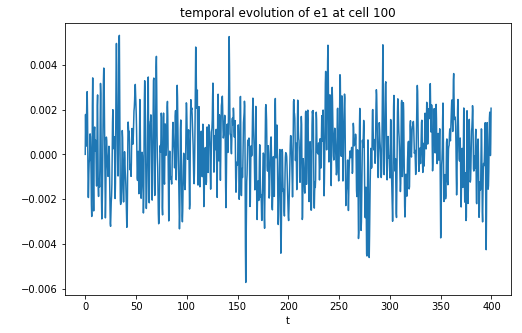Next, in the following two cells, we are going to plot $\omega(k)$. This is generated by taking $E_1(x_1,t)$ and Fourier analyzing in both position and time.

• $\omega(k)$ with wavenumber in units of [k] = $\omega_{pe}/c$:
dirname = 'v02'
osiris.plot_wk(rundir=dirname, wlim=[0,2], klim=[0,25], vth = 0.02, vmin=-1, vmax=3)
osiris.plot_wk(rundir=dirname, wlim=[0,2], klim=[0,25], vth = 0.02, vmin=-1, vmax=3, show_theory=True)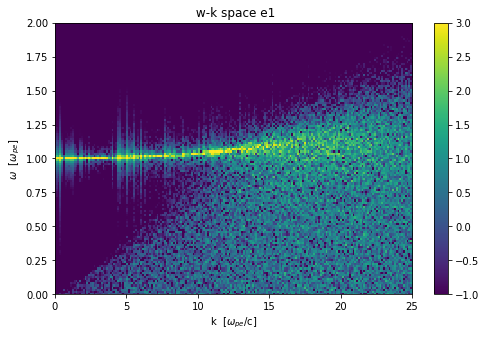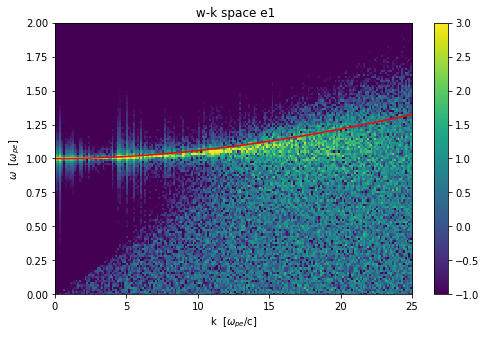• $\omega(k)$ with wavenumber in units of [k] = $\lambda_{De}$:
dirname = 'v02'
osiris.plot_wk(rundir=dirname, wlim=[0,2], klim=[0,0.5], vth = 0.02, vmin=-2, vmax=3, show_theory=True, debye=True)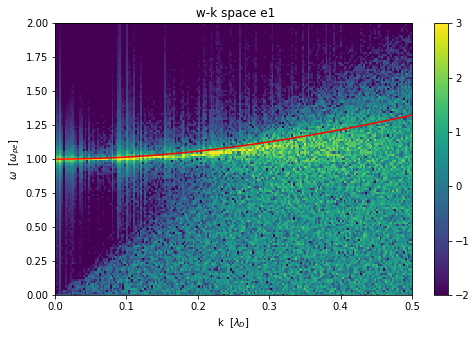### 3.1. I would like you to think about units:

• What do you think are the natural units for $\omega$?

We are plotting them in what are called normalized units (not inverse time or inverse distance). We use two choices ($\omega/\omega_p$ and $kc/\omega_p$) and ($\omega/\omega_p$ and $kv_{th}/\omega_p = k\lambda_D$).

We also plot the theory curve:

• Does it make sense?
• Why do you think it agrees better for smaller wave numbers?
• At what wave number does the theory plot stop working as well?
• Can you explain why this happens?

## 4. Run a case in which $vth_1=vth_2=vth_3=0.05 c$.

# vth/c = 0.05
dirname = 'v05'
osiris.runosiris(rundir=dirname,inputfile='v05.txt')

Make $\omega(k)$ plots for this case by running the cells below.

• $\omega(k)$ with wavenumber in units of [k] = $\omega_{pe}/c$:
dirname = 'v05'
osiris.plot_wk(rundir=dirname, wlim=[0,2], klim=[0,25], vth = 0.05, vmin=-1, vmax=3)
osiris.plot_wk(rundir=dirname, wlim=[0,2], klim=[0,25], vth = 0.05, vmin=-1, vmax=3, show_theory=True)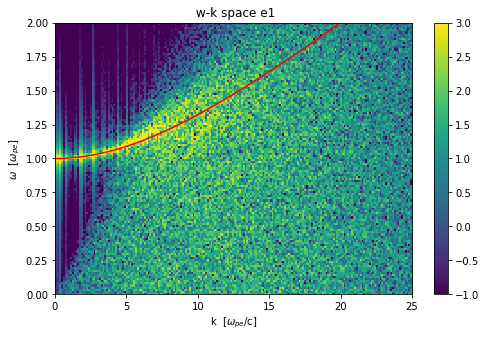• $\omega(k)$ with wavenumber in units of [k] = $\lambda_{De}$:
dirname = 'v05'
osiris.plot_wk(rundir=dirname, wlim=[0,2], klim=[0,0.5], vth = 0.05, vmin=-2, vmax=3, show_theory=True, debye=True)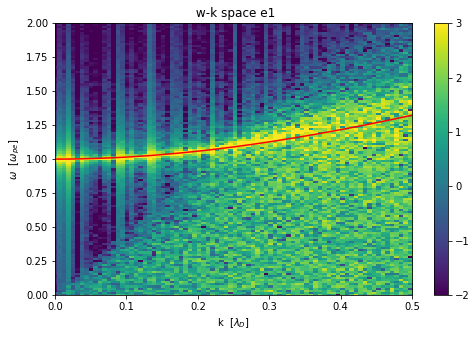### 4.1. Questions

• Do the $\omega(k)$ plots make sense?
• For which normalized units do the plots look similar to case b?

## 5. Run a case in which $vth_1=vth_2=vth_3=0.20 c$.

# vth/c = 0.2
dirname = 'v20'
osiris.runosiris(rundir=dirname,inputfile='v20.txt')

Make $\omega(k)$ plots for this case by running the cells below.

• $\omega(k)$ with wavenumber in units of [k] = $\omega_{pe}/c$:
dirname = 'v20'
osiris.plot_wk(rundir=dirname, wlim=[0,2], klim=[0,25], vth = 0.20, vmin=0, vmax=5)
osiris.plot_wk(rundir=dirname, wlim=[0,2], klim=[0,25], vth = 0.20, vmin=0, vmax=5, show_theory=True)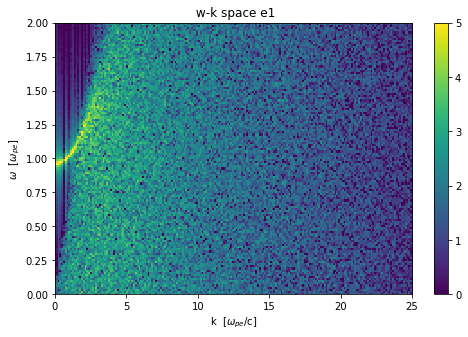• $\omega(k)$ with wavenumber in units of [k] = $\lambda_{De}$:
dirname = 'v20'
osiris.plot_wk(rundir=dirname, wlim=[0,2], klim=[0,0.5], vth = 0.20, vmin=0, vmax=5, show_theory=True, debye=True)### 5.1. Questions

• Do the $\omega(k)$ plots make sense?

• For which normalized units do the plots look similar to case a and b?

• Look closely at $k=0$. The frequency does not agree with theory as well as for cases a and b.

• Is it higher or lower than theory?

• Can you think of a reason why?